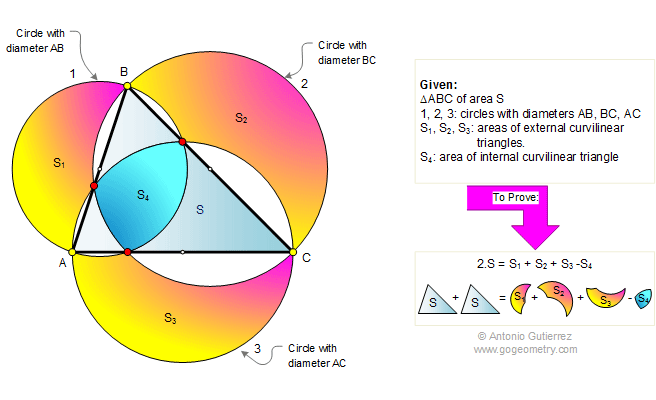Home Geometry Problems Art All Problems 351-360 Semicircle Circular Segment Area of a Triangle View or post a solution
Problem 360. Area of a triangle, Curvilinear triangles, Circles, Diameters.
Level: High School, College, SAT Prep.

In the figure below, ABC is an acute triangle with area S. Circles of diameters AB, BC, and AC form three curvilinear triangles of areas S1, S2, and S3, respectively, and one internal curvilinear triangle of area S4.
Prove that 2S = S1 + S2 + S3 - S4.See Also: Proposed Problem 361. Right triangle, Incircle, Incenter, Tangency points, Angle. Proposed Problem 359. Cyclic quadrilateral, Angle bisector, Rhombus. Proposed Problem 358. Isosceles triangle 80-80-20, Circle, Angles, Congruence.